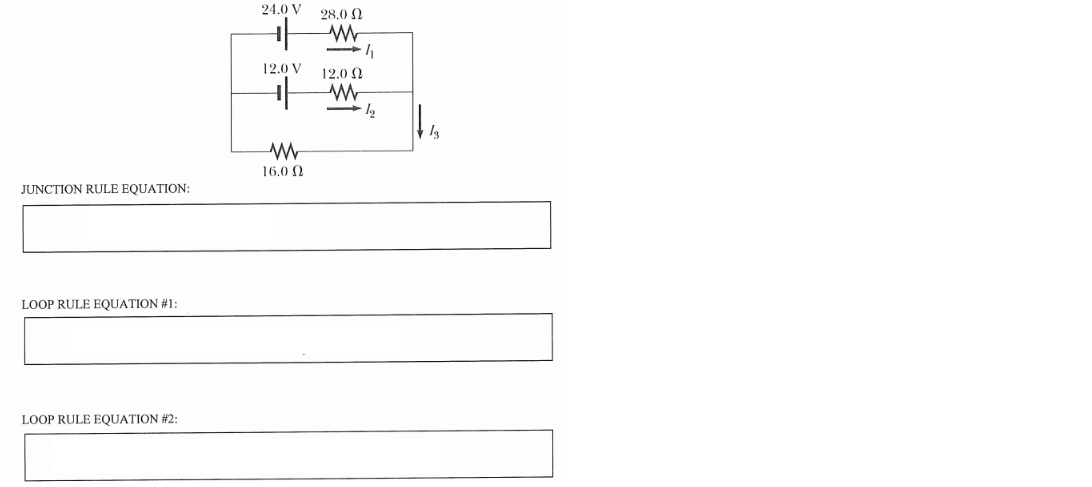# Solution: Present one junction and two loop rule equations that you could solve simultaneously to obtain values for the three currents, I1, I2 and I3, in the dc circuit shown below. Do not solve the equations!

###### Problem

Present one junction and two loop rule equations that you could solve simultaneously to obtain values for the three currents, I1, I2 and I3, in the dc circuit shown below. Do not solve the equations!